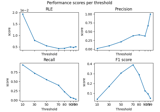# mne.simulation.metrics.spatial_deviation_error#

mne.simulation.metrics.spatial_deviation_error(stc_true, stc_est, src, threshold='50%', per_sample=True)[source]#

Compute the spatial deviation.

The spatial deviation characterizes the spread of the estimate source around the true source.

$SD = \dfrac{\sum_i|s_i|\|r_{i} - r_{true}\|^2}{\sum_i|s_i|}.$

where $$r_{true}$$ is a true dipole position, $$r_i$$ and $$|s_i|$$ denote respectively the position and amplitude of i-th dipole in source estimate.

Threshold is used on estimated source for focusing the metric to strong amplitudes and omitting the low-amplitude values.

Parameters
stc_trueinstance of (Vol|Mixed)SourceEstimate

The source estimates containing correct values.

stc_estinstance of (Vol|Mixed)SourceEstimate

The source estimates containing estimated values e.g. obtained with a source imaging method.

srcinstance of SourceSpaces

The source space on which the source estimates are defined.

threshold

The threshold to apply to source estimates before computing the recall. If a string the threshold is a percentage and it should end with the percent character.

per_samplebool

If True the metric is computed for each sample separately. If False, the metric is spatio-temporal.

Returns
metricfloat | array, shape (n_times,)

The metric. float if per_sample is False, else array with the values computed for each time point.

Notes

These metrics are documented in 1 and 2.

New in v1.2.

References

1

Matti Stenroos and Olaf Hauk. Minimum-norm cortical source estimation in layered head models is robust against skull conductivity error. NeuroImage, 81:265–272, November 2013. doi:10.1016/j.neuroimage.2013.04.086.

2

Fa-Hsuan Lin, Thomas Witzel, Seppo P. Ahlfors, Steven M. Stufflebeam, John W. Belliveau, and Matti S. Hämäläinen. Assessing and improving the spatial accuracy in MEG source localization by depth-weighted minimum-norm estimates. NeuroImage, 31(1):160–171, 2006. doi:10.1016/j.neuroimage.2005.11.054.

## Examples using mne.simulation.metrics.spatial_deviation_error#Compare simulated and estimated source activity

Compare simulated and estimated source activity